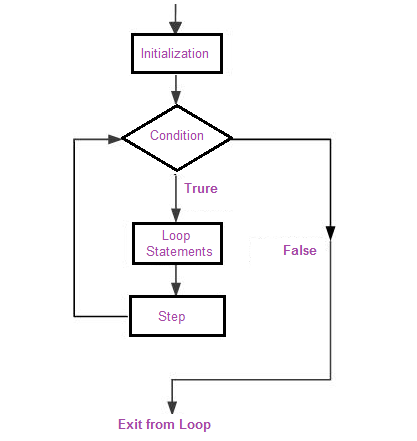# for loop in Java

There are many situation when you want to execute a block of statements several number of times in your applications. Loops can execute a block of code a number of times. The for loop in Java is useful for iterating over arrays and for sequential processing. Syntax:
for (initialization; termination; increment) { statement(s) }
initialization: is executed before the loop (the code block) starts. termination: defines the condition for running the loop (the code block). increment: is executed each time after the loop (the code block) has been executed.The for loop initialize the value before the first step. Then checking the condition against the current value of variable and execute the loop statement and then perform the step taken for each execution of loop body. For-loops are also typically used when the number of iterations is known before entering the loop. For-loops are the shorthand way to make loops when the number of iterations is known, as every for-loop could be written as a while-loop. Example:
int counter = 4; for (int cnt = 1; cnt < = counter; cnt++) { System.out.println("Current value of cnt is: " + cnt); }

The initializer declares and initializes a local loop variable, cnt, that maintains a count of the iterations of the loop. The loop will execute four(4) times because we set the condition cnt is less than or equal to counter.

for (int cnt = 1; cnt < = counter; cnt++) initialization: int cnt = 1 Initialize the variable cnt as 1, that is when the loop starts the value of cnt is set as 1 termination: i < = counter Set the condition i < =counter , that is the loop will execute up to when the value of cnt < = 4 (four times) increment: cnt++ Set the step for each execution of loop block as cnt++ ( cnt = cnt +1)

The output of the code as follows :

Current value of i is: 1 Current value of i is: 2 Current value of i is: 3 Current value of i is: 4
Full Source
public class TestClass { public static void main(String[] args) { int counter = 4; for (int cnt = 1; cnt < = counter; cnt++) { System.out.println("Current value of cnt is: " + cnt); } } }

### Infinite Loop

All of the expressions of the for loop statements are optional. A loop becomes infinite loop if a condition never becomes false. You can make an endless loop by leaving the conditional expression empty.
for ( ; ; ) { System.out.println("Infinite Loop!!!"); }
In the above code the loop will execute infinite times because there is no initialization , condition and steps .

### Processing Arrays using Loops

An "array" is a way to store a collection of "elements" . It would be quite difficult to process each of the array's elements individually. The for statement also has another form designed for iteration through Collections and arrays, it can be used to make your loops more compact and easy to read. The common approach is to use a for loop with a variable used to keep track of the index number and iterate through the entire array. The following program uses the for to loop through the array:
public class TestClass { public static void main(String[] args) { int[] arr = {2,4,8,16}; for(int i=0; i < arr.length; i++){ System.out.println(arr[i]); } } }
Output:
2 4 8 16

Check with the following enhanced for loop is used to print the elements of the array.

public class TestClass { public static void main(String[] args) { int[] arr = {2,4,8,16}; for(int i:arr){ System.out.println(i); } } }
Output:
2 4 8 16
In the above code, value of i is first initialised to arr i.e. 2 and the body of the for loop is executed which will causes the integer 3 to be printed on the screen. Next arr i.e. 4 is assigned to x and the body executed again. In this way the loop continues until all the elements are printed. Here, we can see the enhanced for loop is used to iterate through not just an array, but a collection in general.